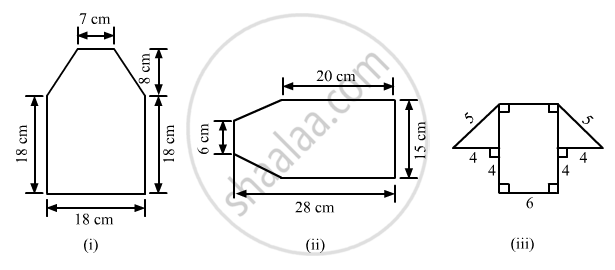Advertisement Remove all ads

# Find the Area Enclosed by Each of the Following Figures [Fig. 20.49 (I)-(Iii)] as the Sum of the Areas of a Rectangle and a Trapezium: - Mathematics

Match the Columns
Sum

Find the area enclosed by each of the following figures [Fig. 20.49 (i)-(iii)] as the sum of the areas of a rectangle and a trapezium:Advertisement Remove all ads

#### Solution$(i)$
The given figure can be divided into a rectangle and a trapezium as shown below:
From the above firgure:
Area of the complete figure = (Area of square ABCF)+(Area of trapezium CDEF)
$=(AB\times BC)+[\frac{1}{2}\times(FC+ED)\times(\text{ Distance between FC and ED })]$
$=(18\times18)+[\frac{1}{2}\times(18+7)\times(8)]$
$=324+100$
${=424 cm}^2$
$(ii)$
The given figure can be divided in the following manner:\]
From the above figure:
AB = AC-BC=28-20=8 cm
So that area of the complete figure = (area of rectangle BCDE)+(area of trapezium ABEF)
$=(BC\times CD)+[\frac{1}{2}\times(BE+AF)\times(AB)]$
$=(20\times15)+[\frac{1}{2}\times(15+6)\times(8)]$
$=300+84$
${=384 cm}^2$

$(iii)$
The given figure can be divided in the following manner:

From the above figure:
EF = AB = 6 cm
Now, using the Pythagoras theorem in the right angle triangle CDE:
$5^2 {= 4}^2 {+CE}^2$
${CE}^2 = 25-16=9$
$CE =\sqrt{9}= 3 cm$
$\text{ And, }GD=GH+HC+CD=4+6+4=14 cm$
$\therefore\text{ Area of the complete figure }= (\text{ Area of rectangle ABCH })+(\text{ Area of trapezium GDEF })$
$=(AB\times BC)+[\frac{1}{2}\times(GD+EF)\times(CE)]$
$=(6\times4)+[\frac{1}{2}\times(14+6)\times(3)]$
$=24+30$
${=54 cm}^2$

Is there an error in this question or solution?
Advertisement Remove all ads

#### APPEARS IN

RD Sharma Class 8 Maths
Chapter 20 Mensuration - I (Area of a Trapezium and a Polygon)
Exercise 20.3 | Q 2 | Page 28
Advertisement Remove all ads

#### Video TutorialsVIEW ALL 

Advertisement Remove all ads
Share
Notifications

View all notifications

Forgot password?
Course